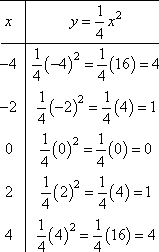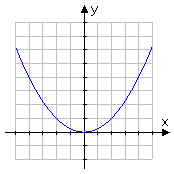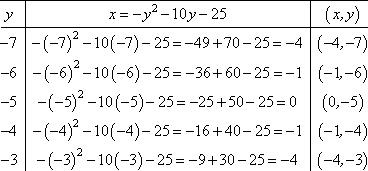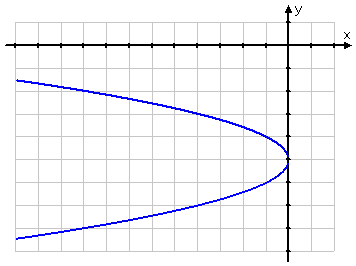Search

Conics: Parabolas:
Finding Information from the Equation
(page 2 of 4)

Sections: Introduction, Finding information from the equation, Finding the equation from information, Word problems & Calculators

• Graph x2 = 4y and state the vertex, focus, axis of symmetry, and directrix.
• This is the same graphing that I've done in the past: y = (1/4)x2. So I'll do the graph as usual:The vertex is obviously at the origin, but I need to "show" this "algebraically" by rearranging the given equation into the conics form:

x2 = 4y Copyright © Elizabeth Stapel 2010-2011 All Rights Reserved
(x  0)2 = 4(y  0)

This rearrangement "shows" that the vertex is at (h, k) = (0, 0). The axis of symmetry is the vertical line right through the vertex: x = 0. (I can always check my graph, if I'm not sure about this.) The focus is "p" units from the vertex. Since the focus is "inside" the parabola and since this is a "right side up" graph, the focus has to be above the vertex.

From the conics form of the equation, shown above, I look at what's multiplied on the unsquared part and see that 4p = 4, so p = 1. Then the focus is one unit above the vertex, at (0, 1), and the directrix is the horizontal line y = 1, one unit below the vertex.

vertex: (0, 0); focus: (0, 1); axis of symmetry: x = 0; directrix: y = 1

• Graph y2 + 10y + x + 25 = 0, and state the vertex, focus, axis of symmetry, and directrix.
• Since the y is squared in this equation, rather than the x, then this is a "sideways" parabola. To graph, I'll do my T-chart backwards, picking y-values first and then finding the corresponding x-values for x = y2  10y  25:To convert the equation into conics form and find the exact vertex, etc, I'll need to convert the equation to perfect-square form. In this case, the squared side is already a perfect square, so:

y2 + 10y + 25 = x
(y + 5)2 = 1(x  0)

This tells me that 4p = 1, so p = 1/4. Since the parabola opens to the left, then the focus is 1/4 units to the left of the vertex. I can see from the equation above that the vertex is at (h, k) = (0, 5), so then the focus must be at (1/4, 5). The parabola is sideways, so the axis of symmetry is, too. The directrix, being perpendicular to the axis of symmetry, is then vertical, and is 1/4 units to the right of the vertex. Putting this all together, I get:

vertex: (0, 5); focus: (1/4, 5); axis of symmetry: y = 5; directrix: x = 1/4

• Find the vertex and focus of y2 + 6y + 12x  15 = 0

The y part is squared, so this is a sideways parabola. I'll get the y stuff by itself on one side of the equation, and then complete the square to convert this to conics form.

y2 + 6y  15 = 12x
y
2 + 6y + 9  15 = 12x + 9

(y + 3)2  15 = 12x + 9

(y + 3)2 = 12x + 9 + 15 = 12x + 24

(y + 3)2 = 12(x  2)

(y  (3))2 = 4(3)(x  2)

Then the vertex is at (h, k) = (2, 3) and the value of p is 3. Since y is squared and p is negative, then this is a sideways parabola that opens to the left. This puts the focus 3 units to the left of the vertex.

vertex: (2, 3); focus: (1, 3)

<< Previous  Top  |  1 | 2 | 3 | 4  |  Return to Index  Next >>

 Cite this article as: Stapel, Elizabeth. "Conics: Parabolas: Finding Information From the Equation." Purplemath.     Available fromhttps://www.purplemath.com/modules/parabola2.htm.     Accessed [Date] [Month] 2016

Reviews of
Internet Sites:
Free Help
Practice
Et Cetera

Study Skills Survey

Tutoring from Purplemath
Find a local math tutor

 Copyright © 2010-2012  Elizabeth Stapel   |   About   |   Terms of Use Feedback   |   Error?